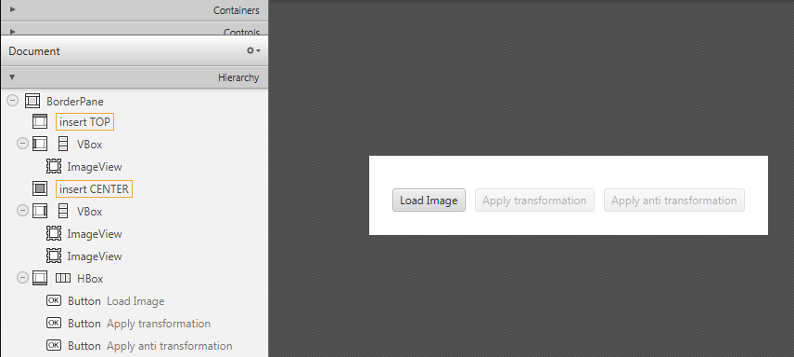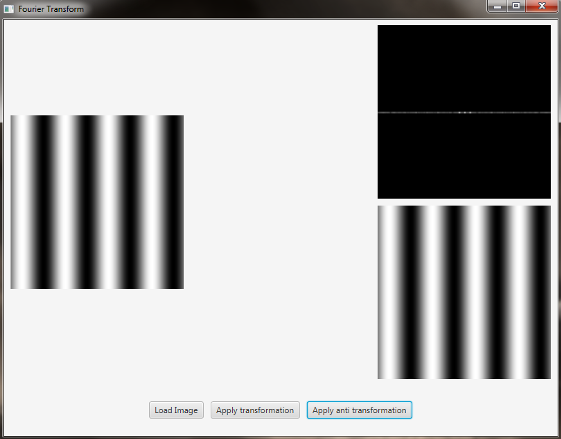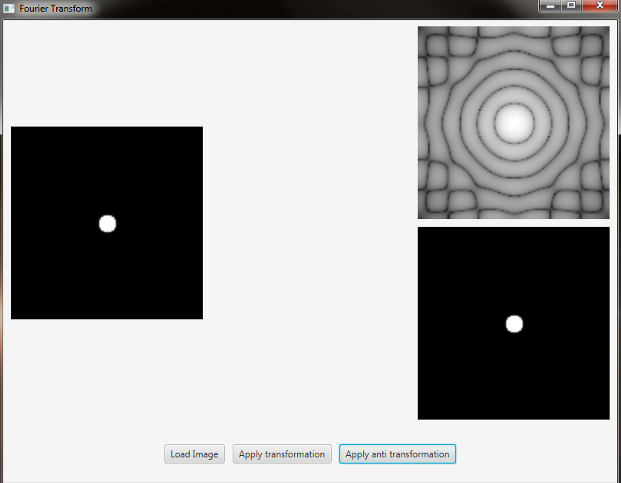# Fourier Transform¶

Note

We assume that by now you have already read the previous tutorials. If not, please check previous tutorials at http://opencv-java-tutorials.readthedocs.org/en/latest/index.html. You can also find the source code and resources at https://github.com/opencv-java/

## Goal¶

In this tutorial we are going to create a JavaFX application where we can load a picture from our file system and apply to it the DFT and the inverse DFT.

## What is the Fourier Transform?¶

The Fourier Transform will decompose an image into its sinus and cosines components. In other words, it will transform an image from its spatial domain to its frequency domain. The result of the transformation is complex numbers. Displaying this is possible either via a real image and a complex image or via a magnitude and a phase image. However, throughout the image processing algorithms only the magnitude image is interesting as this contains all the information we need about the images geometric structure. For this tutorial we are going to use basic gray scale image, whose values usually are between zero and 255. Therefore the Fourier Transform too needs to be of a discrete type resulting in a Discrete Fourier Transform (DFT). The DFT is the sampled Fourier Transform and therefore does not contain all frequencies forming an image, but only a set of samples which is large enough to fully describe the spatial domain image. The number of frequencies corresponds to the number of pixels in the spatial domain image, i.e. the image in the spatial and Fourier domain are of the same size.

## What we will do in this tutorial¶

In this guide, we will:
• Load an image from a file chooser.
• Apply the DFT to the loaded image and show it.
• Apply the iDFT to the transformed image and show it.

## Getting Started¶

Let’s create a new JavaFX project. In Scene Builder set the windows element so that we have a Border Pane with:

• on the LEFT an ImageView to show the loaded picture:
<ImageView fx:id="originalImage" />

• on the RIGHT two ImageViews one over the other to display the DFT and the iDFT;
<ImageView fx:id="transformedImage" />
<ImageView fx:id="antitransformedImage" />

• on the BOTTOM three buttons, the first one to load the picture, the second one to apply the DFT and show it, and the last one to apply the anti-transform and show it.
<Button alignment="center" text="Load Image" onAction="#loadImage"/>
<Button fx:id="transformButton" alignment="center" text="Apply transformation" onAction="#transformImage" disable="true" />
<Button fx:id="antitransformButton" alignment="center" text="Apply anti transformation" onAction="#antitransformImage" disable="true" />


The gui will look something like this one:First of all you need to add to your project a folder resources with two files in it. One of them is a sine function and the other one is a circular aperture. In the Controller file, in order to load the image to our program, we are going to use a filechooser:

private FileChooser fileChooser;


When we click the load button we have to set the initial directory of the FC and open the dialog. The FC will return the selected file:

File file = new File("./resources/");
this.fileChooser.setInitialDirectory(file);
file = this.fileChooser.showOpenDialog(this.main.getStage());


Once we’ve loaded the file we have to make sure that it’s going to be in grayscale and display the image into the image view:

this.image = Imgcodecs.imread(file.getAbsolutePath(), Imgcodecs.CV_LOAD_IMAGE_GRAYSCALE);
this.originalImage.setImage(this.mat2Image(this.image));


## Applying the DFT¶

First of all expand the image to an optimal size. The performance of a DFT is dependent of the image size. It tends to be the fastest for image sizes that are multiple of the numbers two, three and five. Therefore, to achieve maximal performance it is generally a good idea to pad border values to the image to get a size with such traits. The getOptimalDFTSize() returns this optimal size and we can use the copyMakeBorder() function to expand the borders of an image:

int addPixelRows = Core.getOptimalDFTSize(image.rows());


The appended pixels are initialized with zeros.

The result of the DFT is complex so we have to make place for both the complex and the real values. We store these usually at least in a float format. Therefore we’ll convert our input image to this type and expand it with another channel to hold the complex values:

padded.convertTo(padded, CvType.CV_32F);
Core.merge(this.planes, this.complexImage);


Now we can apply the DFT and then get the real and the imaginary part from the complex image:

Core.dft(this.complexImage, this.complexImage);
Core.split(complexImage, newPlanes);
Core.magnitude(newPlanes.get(0), newPlanes.get(1), mag);


Unfortunately the dynamic range of the Fourier coefficients is too large to be displayed on the screen. To use the gray scale values for visualization we can transform our linear scale to a logarithmic one:

Core.add(Mat.ones(mag.size(), CVType.CV_32F), mag);
Core.log(mag, mag);


Remember, that at the first step, we expanded the image? Well, it’s time to throw away the newly introduced values. For visualization purposes we may also rearrange the quadrants of the result, so that the origin (zero, zero) corresponds with the image center:

image = image.submat(new Rect(0, 0, image.cols() & -2, image.rows() & -2));
int cx = image.cols() / 2;
int cy = image.rows() / 2;

Mat q0 = new Mat(image, new Rect(0, 0, cx, cy));
Mat q1 = new Mat(image, new Rect(cx, 0, cx, cy));
Mat q2 = new Mat(image, new Rect(0, cy, cx, cy));
Mat q3 = new Mat(image, new Rect(cx, cy, cx, cy));

Mat tmp = new Mat();
q0.copyTo(tmp);
q3.copyTo(q0);
tmp.copyTo(q3);

q1.copyTo(tmp);
q2.copyTo(q1);
tmp.copyTo(q2);


Now we have to normalize our values by using the normalize() function in order to transform the matrix with float values into a viewable image form:

Core.normalize(mag, mag, 0, 255, Core.NORM_MINMAX);


The last step is to show the magnitude image in the ImageView:

this.transformedImage.setImage(this.mat2Image(magnitude));


## Applying the inverse DFT¶

To apply the inverse DFT we simply use the idft() function, extract the real values from the complex image with the split() function, and normalize the result with normalize():

Core.idft(this.complexImage, this.complexImage);
Mat restoredImage = new Mat();
Core.split(this.complexImage, this.planes);
Core.normalize(this.planes.get(0), restoredImage, 0, 255, Core.NORM_MINMAX);


Finally we can show the result on the proper ImageView:

this.antitransformedImage.setImage(this.mat2Image(restoredImage));


## Analyzing the results¶

• sinfunction.pngThe image is a horizontal sine of 4 cycles. Notice that the DFT just has a single component, represented by 2 bright spots symmetrically placed about the center of the DFT image. The center of the image is the origin of the frequency coordinate system. The x-axis runs left to right through the center and represents the horizontal component of frequency. The y-axis runs bottom to top through the center and represents the vertical component of frequency. There is a dot at the center that represents the (0,0) frequency term or average value of the image. Images usually have a large average value (like 128) and lots of low frequency information so FT images usually have a bright blob of components near the center. High frequencies in the horizontal direction will cause bright dots away from the center in the horizontal direction.

• circle.pngIn this case we have a circular aperture, and what is the Fourier transform of a circular aperture? The diffraction disk and rings. A large aperture produces a compact transform, instead a small one produces a larger Airy pattern; thus the disk is greater if aperture is smaller; according to Fourier properties, from the center to the middle of the first dark ring the distance is (1.22 x N) / d; in this case N is the size of the image, and d is the diameter of the circle. An Airy disk is the bright center of the diffraction pattern created from a circular aperture ideal optical system; nearly half of the light is contained in a diameter of 1.02 x lamba x f_number.

The source code of the entire tutorial is available on GitHub.